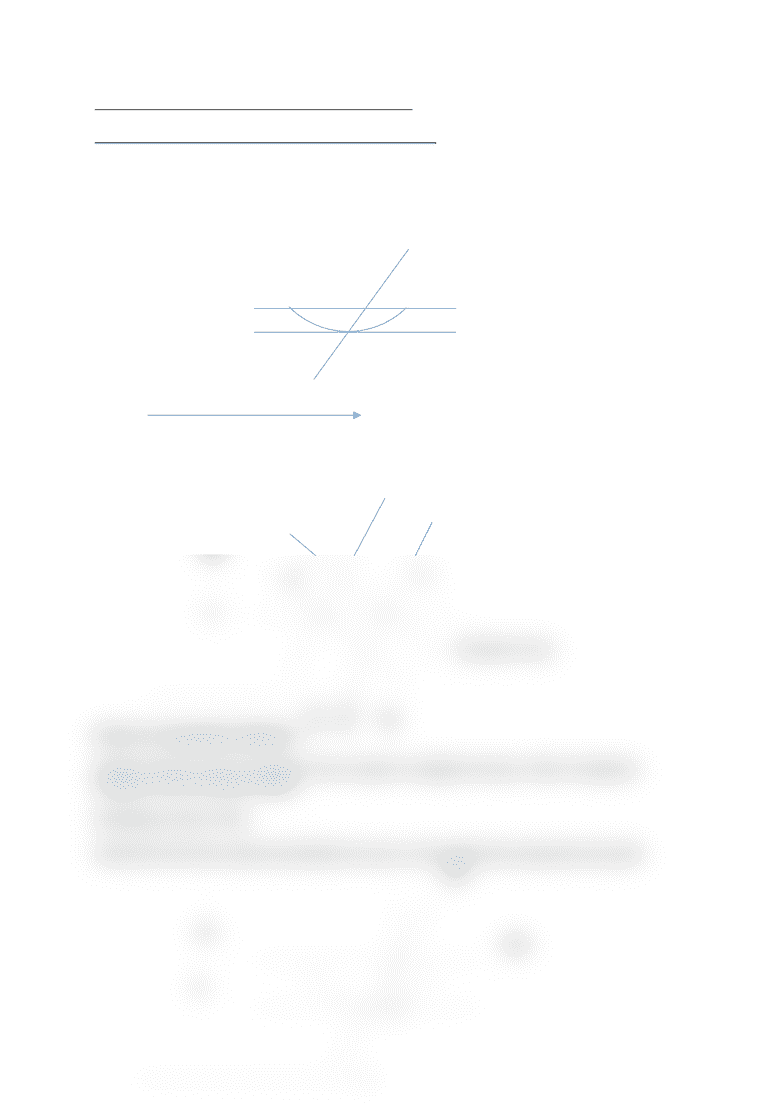# ECON 1116 Lecture Notes - Lecture 9: Marginal Revenue, Perfect Competition, Fixed Cost

126 views2 pages
School
Department
Course
ProfessorECON 1116 Lecture 9:The costs of Production
Short run and long run effects of increase in demand:
A firm begins in long run equilibrium but then an increase in demand raises P leading to SR
profits for the firm over time profits induce entry shifting s to the right reducing P
One Firm
Quantity
Market
Q1 Q2 Q3
Firms in Competitive Markets
Always Produce where marginal Cost is equal to marginal revenue. Most Companies
operate at zero economic profit.
Identifying a firms Profit
Determine this firms profit and identify the area on the graph that represents the profit
Average Total Cost Curve
MC
Price
Profit
Supply
Curve
B
P2
CA
P1
Demand Curve
MC
10
6
MR
Unlock document

This preview shows half of the first page of the document.
Unlock all 2 pages and 3 million more documents.

## Document Summary

Short run and long run effects of increase in demand: A firm begins in long run equilibrium but then an increase in demand raises p leading to sr profits for the firm over time profits induce entry shifting s to the right reducing p. Always produce where marginal cost is equal to marginal revenue. Determine this firms profit and identify the area on the graph that represents the profit. In the above graph of quantity vs price, the firms profit is 4 \$ quantity of 50 units is produced at the price of . In the long run a typical firm earns zero profit. Each firm by definition produces maximum quantity i. e. as much as you can. If you raise your price the buyers will go to another supplier. Shutdown: a short run decision not to produce anything because of market conditions. Exit: a long run decision to leave the market.

## Get access

\$10 USD/m
Billed \$120 USD annuallyHomework Help
Study Guides
Textbook Solutions
Class Notes
Textbook Notes
Booster Class